# RS Aggarwal Solutions for Class 10 Maths Chapter 17 Volume and Surface Area of Solids Exercise 17A

R S Aggarwal Solutions for Class 10 Maths Chapter 17 Volume and Surface Area of Solids, contains solutions for exercise 17A questions. This exercise is all about solving problems on volume and surface area of solids and their combinations. Students can download this exercise PDF from the link given below.

## Download PDF of RS Aggarwal Solutions for Class 10 Maths Chapter 17 Volume and Surface Area of Solids Exercise 17A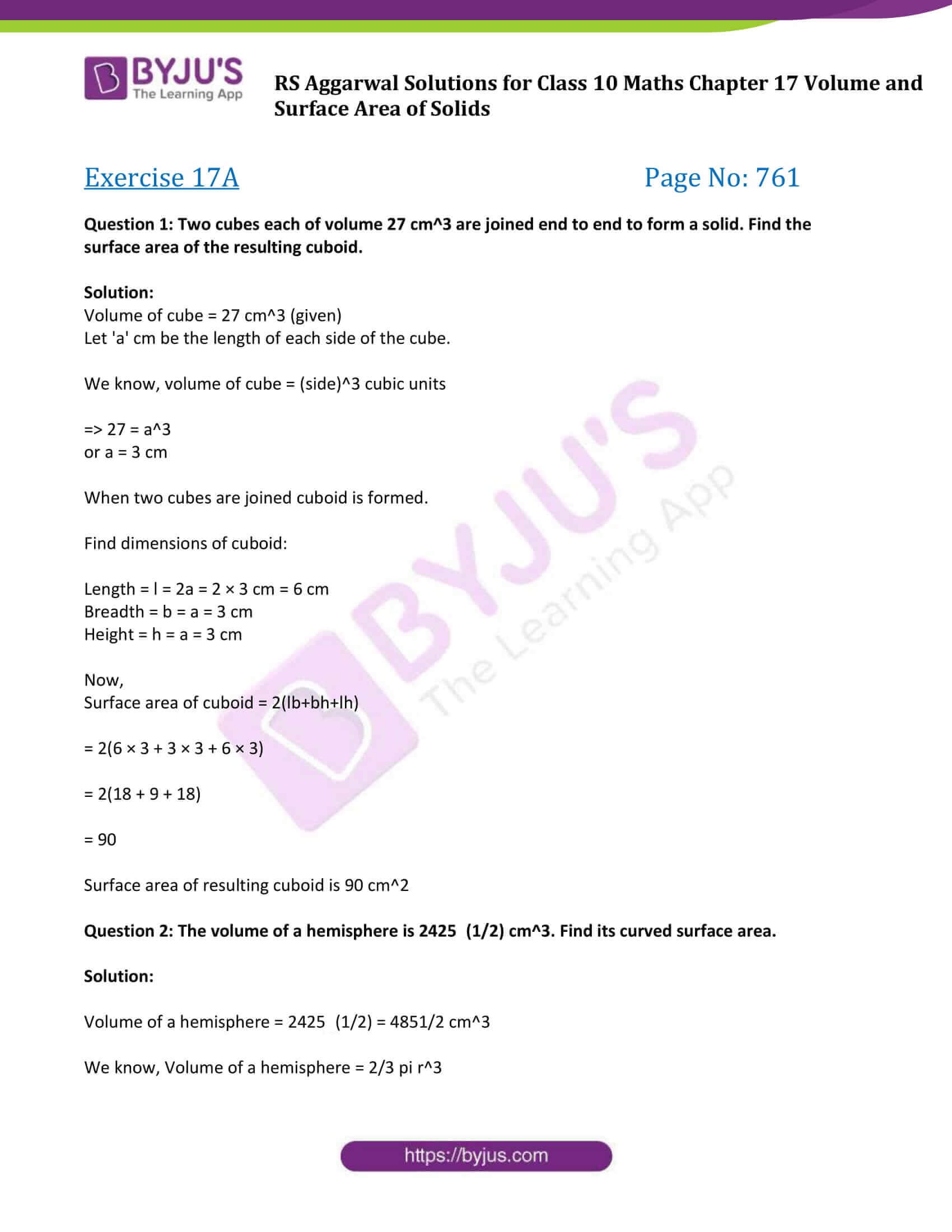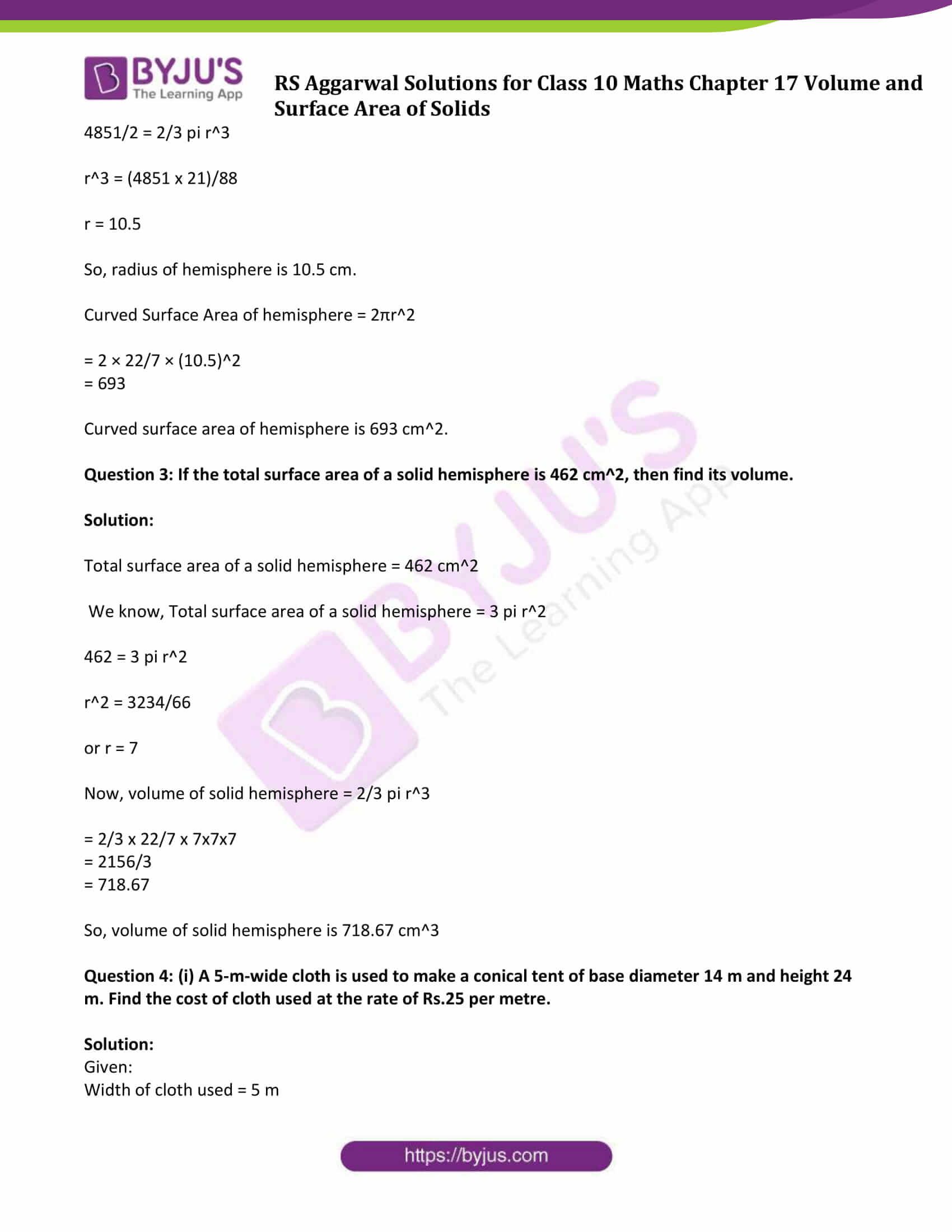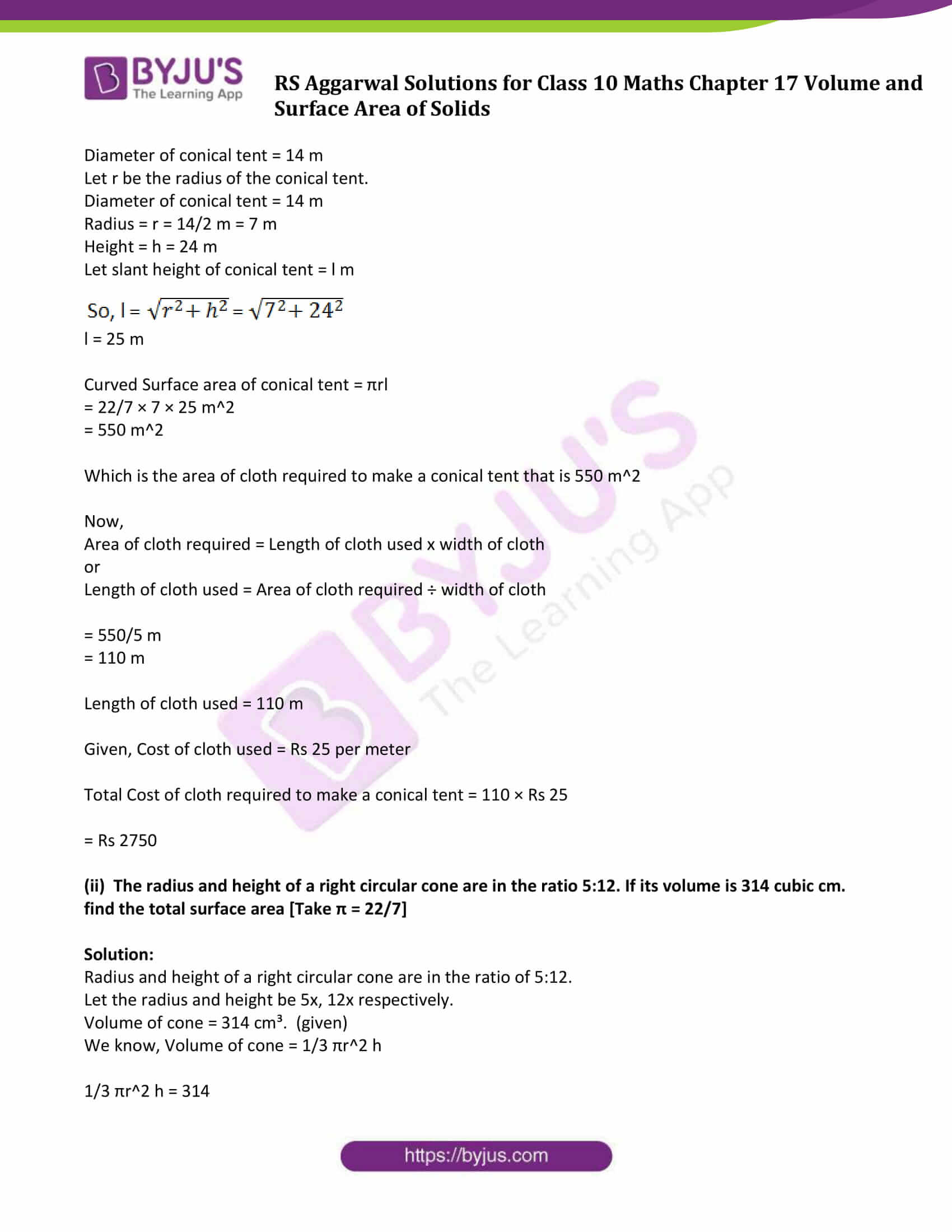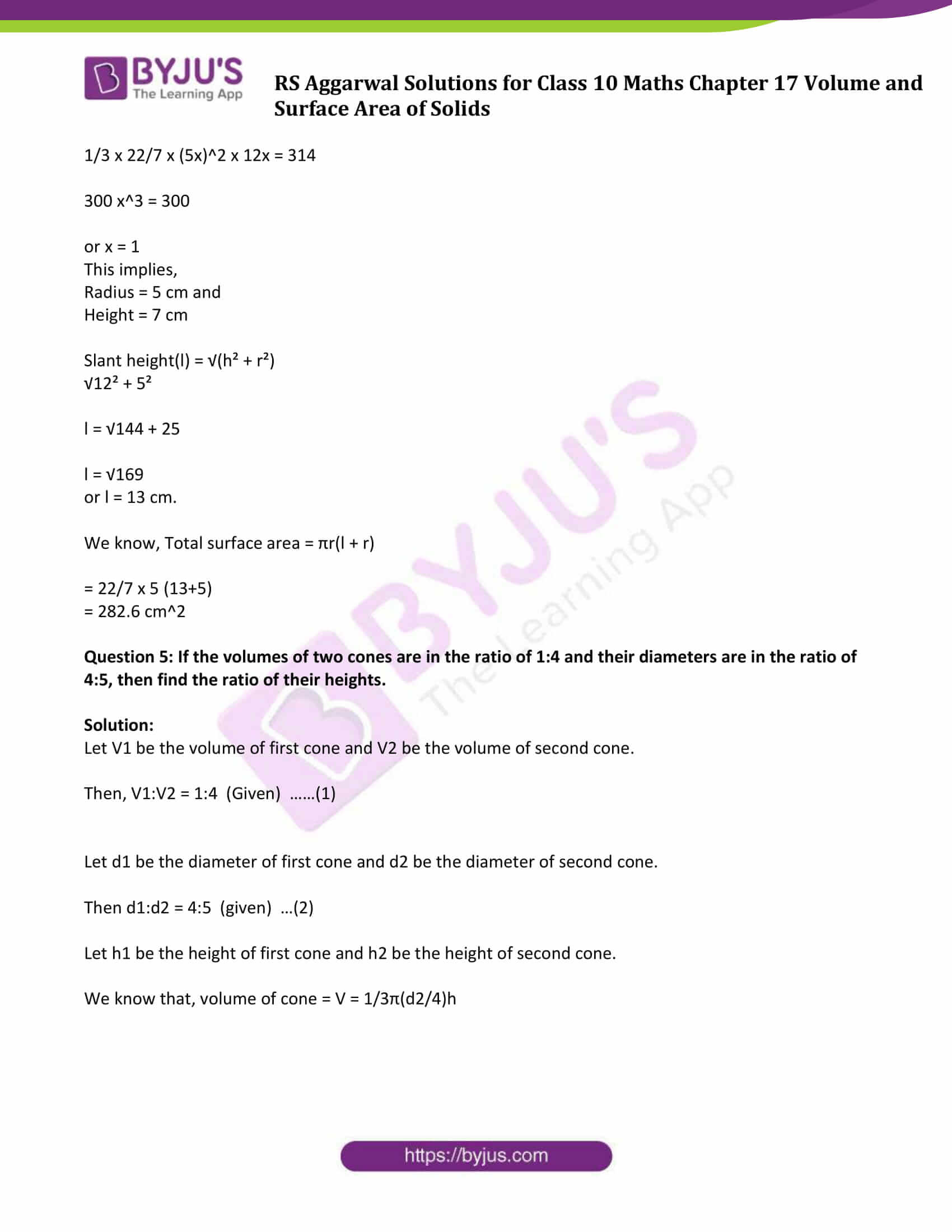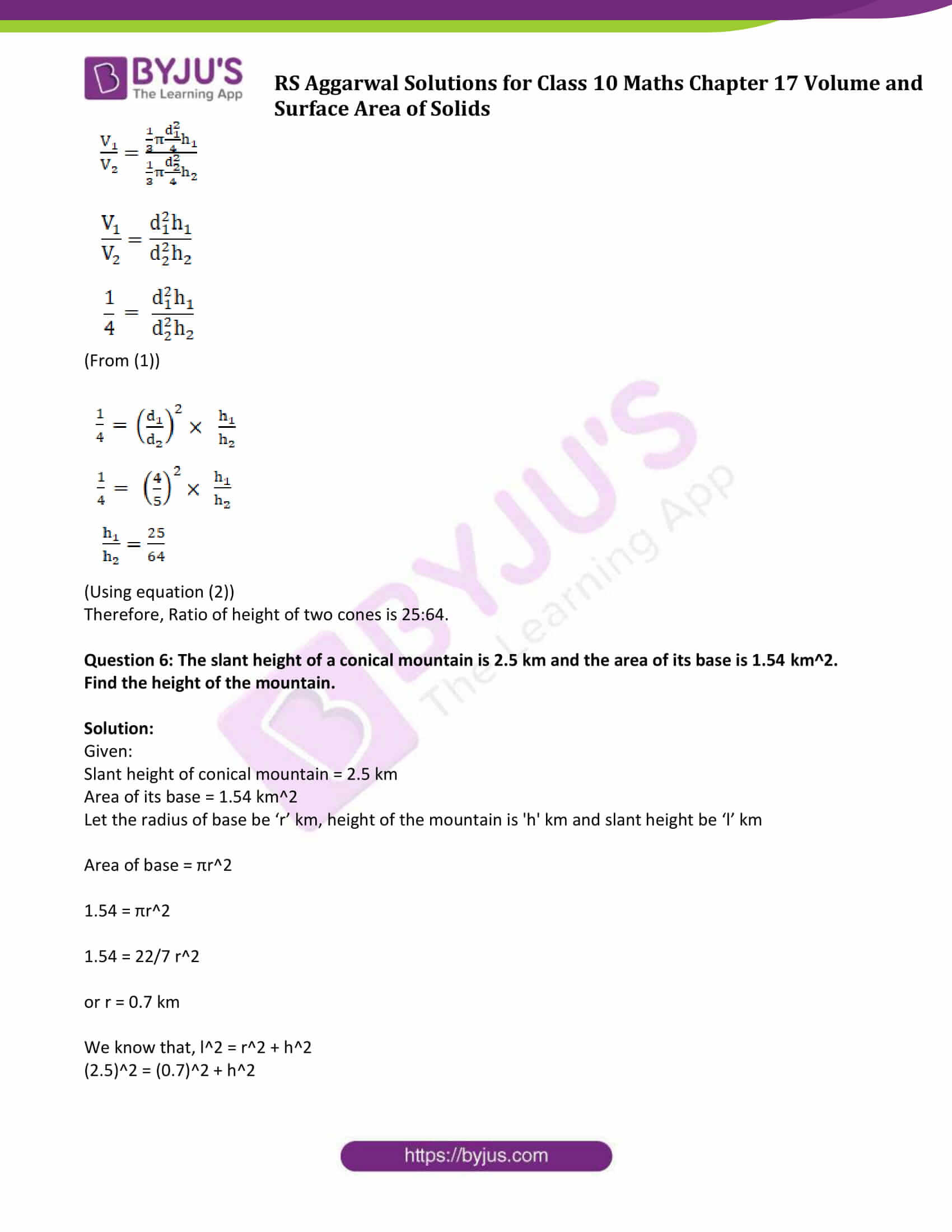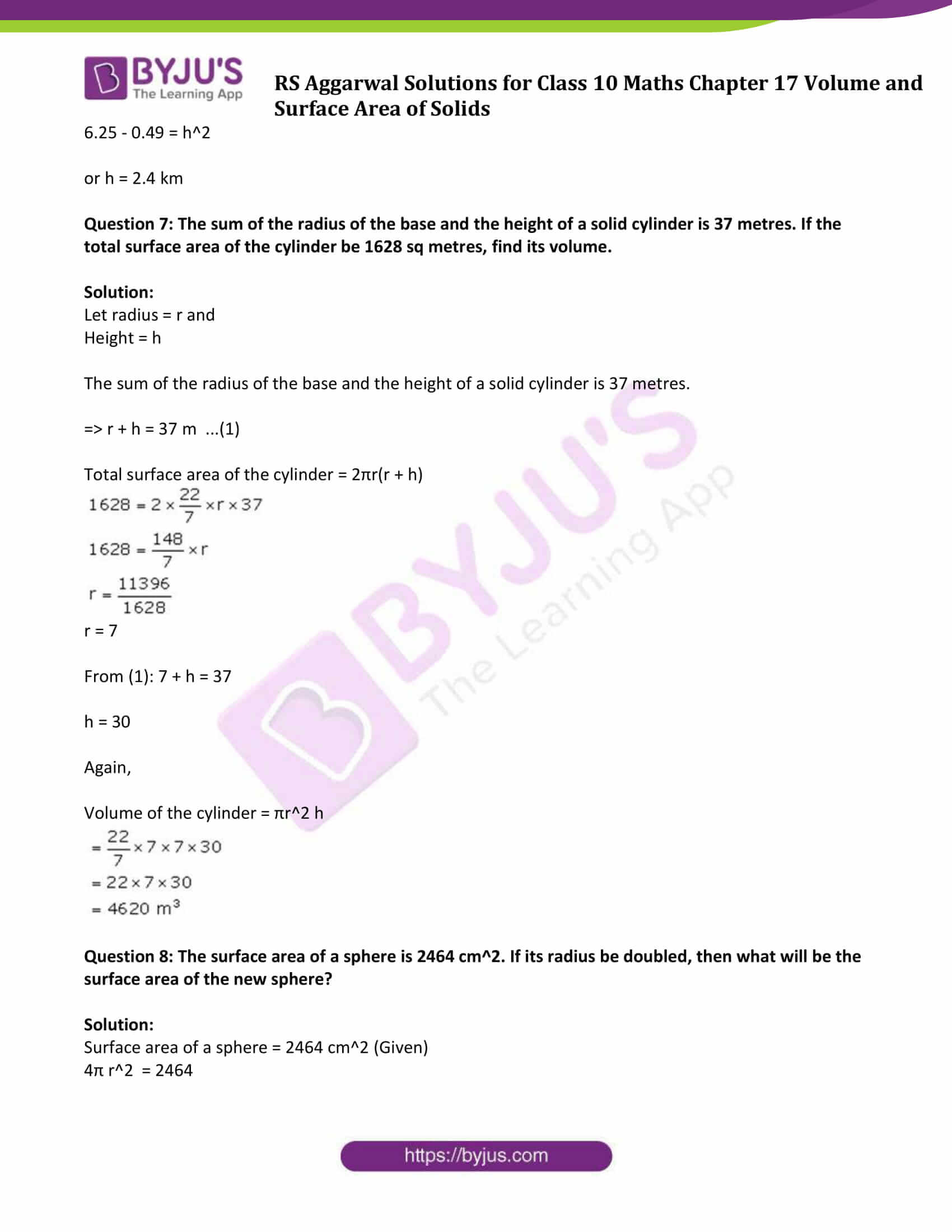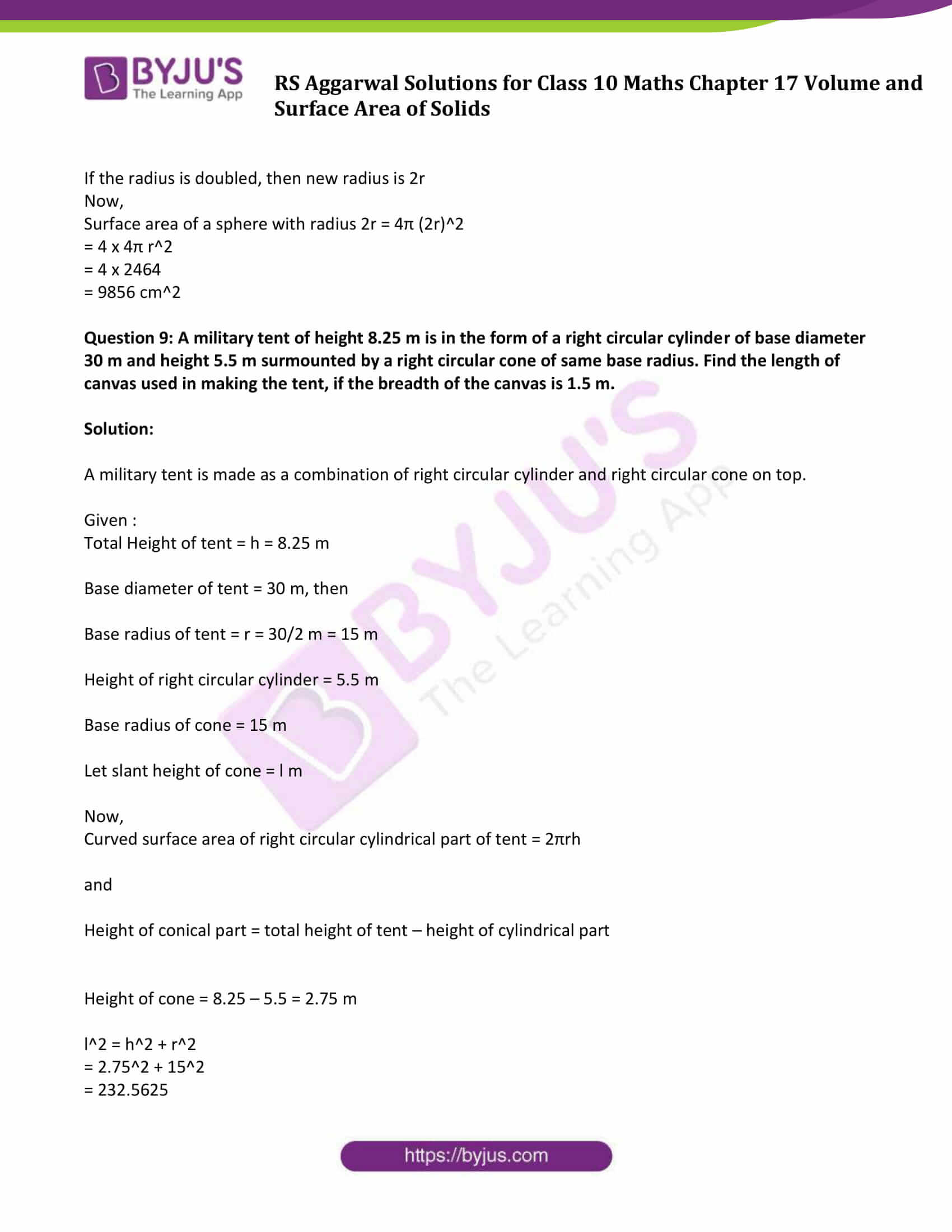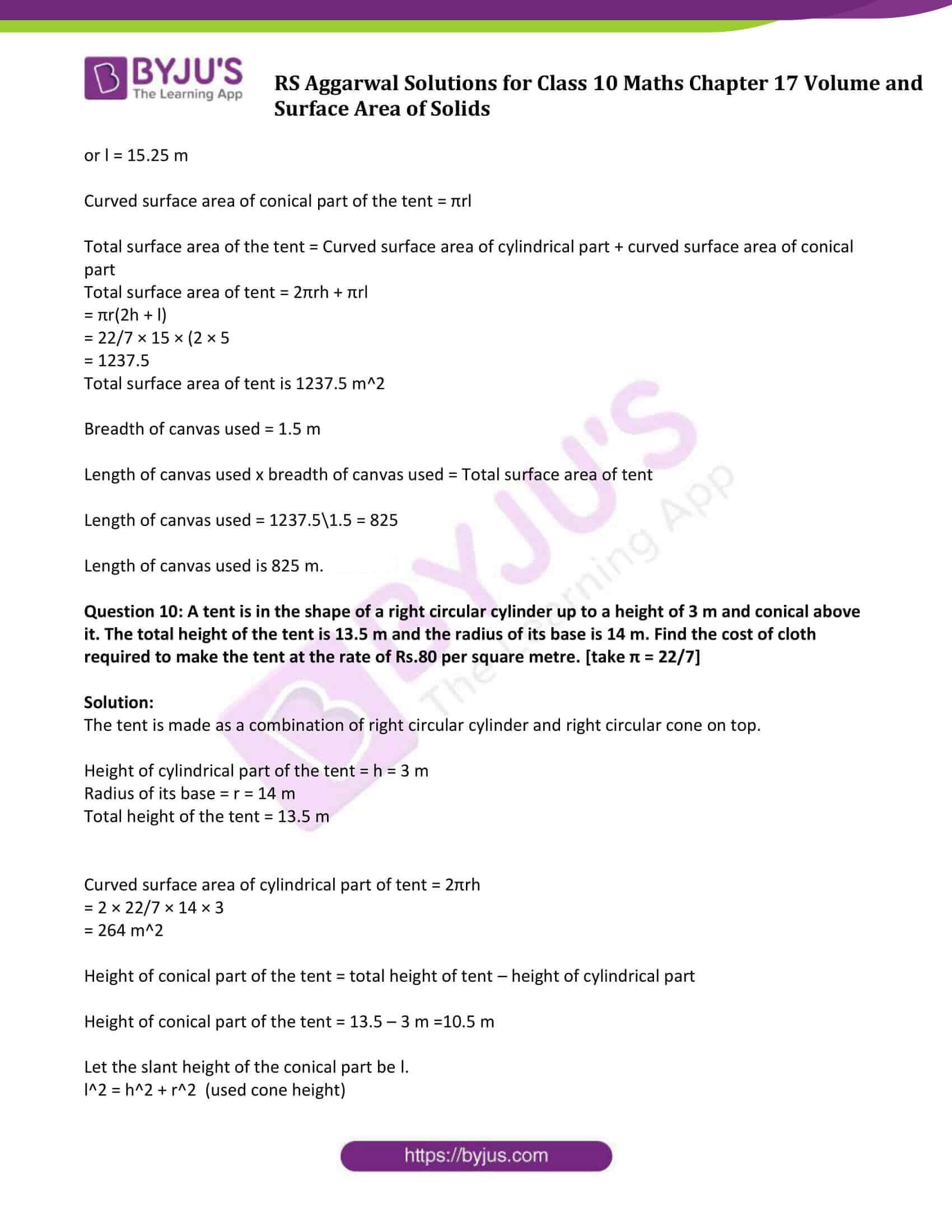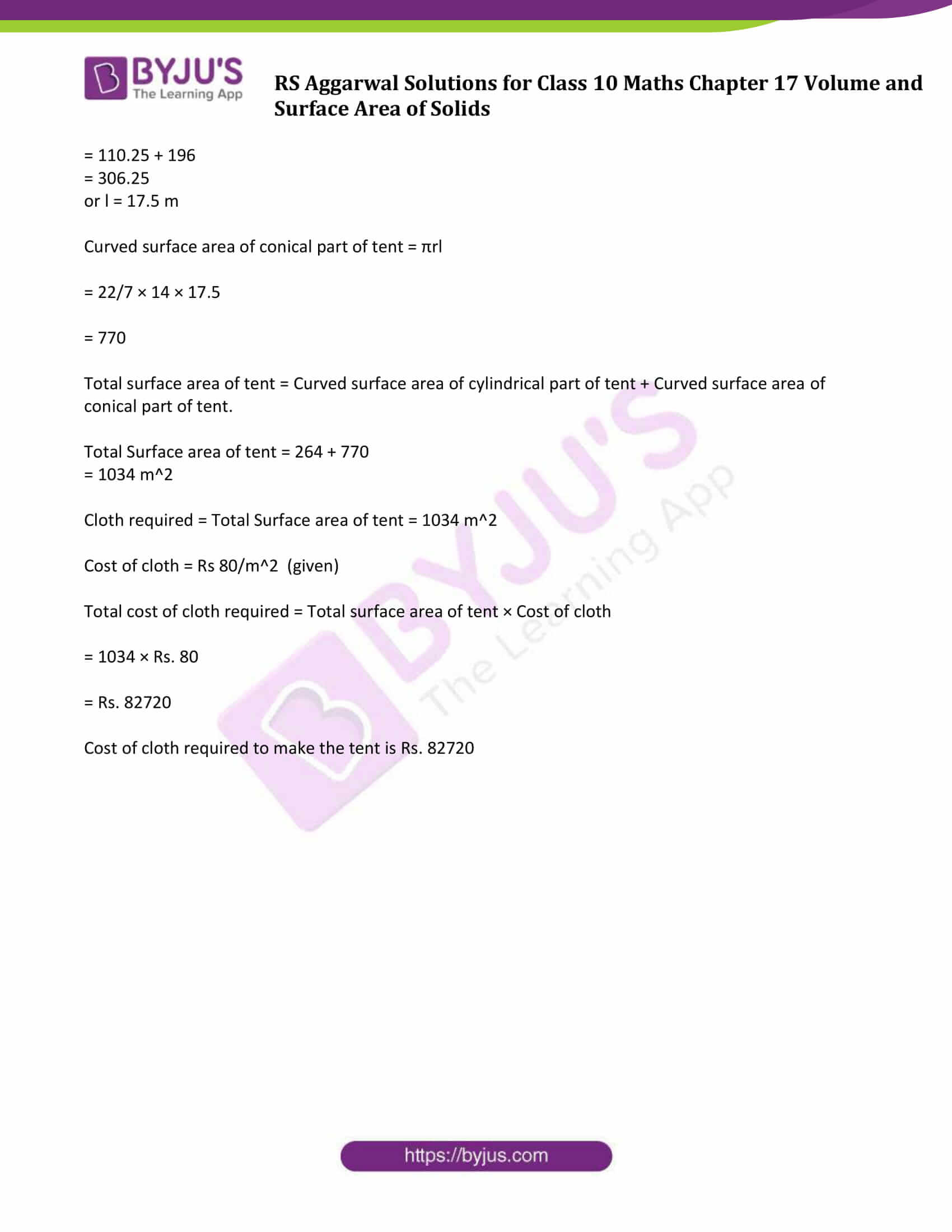### Access other exercise solutions of Class 10 Maths Chapter 17 Volume and Surface Area of Solids

Exercise 17 B Solutions

Exercise 17 C Solutions

Exercise 17 D Solutions

### Access Solutions of Maths RS Aggarwal Class 10 Chapter 17 – Volume and Surface Area of Solids Exercise 17A

Question 1: Two cubes each of volume 27 cm3 are joined end to end to form a solid. Find the surface area of the resulting cuboid.

Solution:

Volume of cube = 27 cm3 (given)

Let ‘a’ cm be the length of each side of the cube.

We know, volume of cube = (side)3 cubic units

⇨ 27 = a3

or a = 3 cm

When two cubes are joined cuboid is formed.

Find dimensions of cuboid:

Length = l = 2a = 2 × 3 cm = 6 cm

Breadth = b = a = 3 cm

Height = h = a = 3 cm

Now,

Surface area of cuboid = 2(lb+bh+lh)

= 2(6 × 3 + 3 × 3 + 6 × 3)

= 2(18 + 9 + 18)

= 90

Surface area of resulting cuboid is 90 cm2

Question 2: The volume of a hemisphere is 2425(1/2) cm3. Find its curved surface area.

Solution:

Volume of a hemisphere = 2425(1/2) = 4851/2 cm3

We know, Volume of a hemisphere = 2/3 pi r3

4851/2 = 2/3 pi r3

r3 = (4851 x 21)/88

r = 10.5

So, radius of hemisphere is 10.5 cm.

Curved Surface Area of hemisphere = 2πr2

= 2 × 22/7 × (10.5)2

= 693

Curved surface area of hemisphere is 693 cm2.

Question 3: If the total surface area of a solid hemisphere is 462 cm2, then find its volume.

Solution:

Total surface area of a solid hemisphere = 462 cm2

We know, Total surface area of a solid hemisphere = 3 pi r2

462 = 3 pi r2

r2 = 3234/66

or r = 7

Now, volume of solid hemisphere = 2/3 pi r3

= 2/3 x 22/7 x 7x7x7

= 2156/3

= 718.67

So, volume of solid hemisphere is 718.67 cm3

Question 4: (i) A 5-m-wide cloth is used to make a conical tent of base diameter 14 m and height 24 m. Find the cost of cloth used at the rate of Rs.25 per metre.

Solution:

Given:

Width of cloth used = 5 m

Diameter of conical tent = 14 m

Let r be the radius of the conical tent.

Diameter of conical tent = 14 m

Radius = r = 14/2 m = 7 m

Height = h = 24 m

Let slant height of conical tent = l m

So , $I = \sqrt{r^{2}+h^{2}} \,=\, \sqrt{7^{2}+24^{2}}$

l = 25 m

Curved Surface area of conical tent = πrl

= 22/7 × 7 × 25 m2

= 550 m2

Which is the area of cloth required to make a conical tent that is 550 m2

Now,

Area of cloth required = Length of cloth used x width of cloth

or

Length of cloth used = Area of cloth required ÷ width of cloth

= 550/5 m

= 110 m

Length of cloth used = 110 m

Given, Cost of cloth used = Rs 25 per meter

Total Cost of cloth required to make a conical tent = 110 × Rs 25

= Rs 2750

(ii) The radius and height of a right circular cone are in the ratio 5:12. If its volume is 314 cubic cm. find the total surface area [Take π = 22/7]

Solution:

Radius and height of a right circular cone are in the ratio of 5:12.

Let the radius and height be 5x, 12x respectively.

Volume of cone = 314 cm³. (given)

We know, Volume of cone = 1/3 πr2 h

1/3 πr2 h = 314

1/3 x 22/7 x (5x)2 x 12x = 314

300 x3 = 300

or x = 1

This implies,

Height = 7 cm

Slant height(l) = √(h² + r²)

√12² + 5²

l = √144 + 25

l = √169

or l = 13 cm.

We know, Total surface area = πr(l + r)

= 22/7 x 5 (13+5)

= 282.6 cm2

Question 5: If the volumes of two cones are in the ratio of 1:4 and their diameters are in the ratio of 4:5, then find the ratio of their heights.

Solution:

Let V1 be the volume of first cone and V2 be the volume of second cone.

Then, V1:V2 = 1:4 (Given) ……(1)

Let d1 be the diameter of first cone and d2 be the diameter of second cone.

Then d1:d2 = 4:5 (given) …(2)

Let h1 be the height of first cone and h2 be the height of second cone.

We know that, volume of cone = V = 1/3π(d2/4)h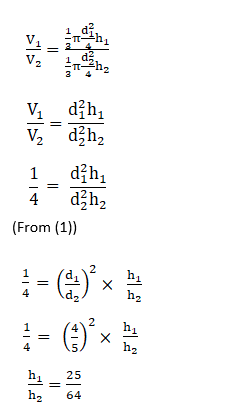(Using equation (2))

Therefore, Ratio of height of two cones is 25:64.

Question 6: The slant height of a conical mountain is 2.5 km and the area of its base is 1.54 km2. Find the height of the mountain.

Solution:

Given:

Slant height of conical mountain = 2.5 km

Area of its base = 1.54 km2

Let the radius of base be ‘r’ km, height of the mountain is ‘h’ km and slant height be ‘l’ km

Area of base = πr2

1.54 = πr2

1.54 = 22/7 r2

or r = 0.7 km

We know that, l2 = r2 + h2

(2.5)2 = (0.7)2 + h2

6.25 – 0.49 = h2

or h = 2.4 km

Question 7: The sum of the radius of the base and the height of a solid cylinder is 37 metres. If the total surface area of the cylinder be 1628 sq metres, find its volume.

Solution:

Height = h

The sum of the radius of the base and the height of a solid cylinder is 37 metres.

⇨ r + h = 37 m …(1)

Total surface area of the cylinder = 2πr(r + h)

1628 = 2 x (22/7) x r x 37

1628 = (148/7) x r

r = 11396/1628

r = 7

From (1): 7 + h = 37

h = 30

Again,

Volume of the cylinder = πr2 h

= (22/7) x 7 x 7 x 30

= 22 x 7 x 30

4620 m3

Question 8: The surface area of a sphere is 2464 cm2. If its radius be doubled, then what will be the surface area of the new sphere?

Solution:

Surface area of a sphere = 2464 cm2 (Given)

4π r2 = 2464

Now,

Surface area of a sphere with radius 2r = 4π (2r)2

= 4 x 4π r2

= 4 x 2464

= 9856 cm2

Question 9: A military tent of height 8.25 m is in the form of a right circular cylinder of base diameter 30 m and height 5.5 m surmounted by a right circular cone of same base radius. Find the length of canvas used in making the tent, if the breadth of the canvas is 1.5 m.

Solution:

A military tent is made as a combination of right circular cylinder and right circular cone on top.

Given :

Total Height of tent = h = 8.25 m

Base diameter of tent = 30 m, then

Base radius of tent = r = 30/2 m = 15 m

Height of right circular cylinder = 5.5 m

Base radius of cone = 15 m

Let slant height of cone = l m

Now,

Curved surface area of right circular cylindrical part of tent = 2πrh

and

Height of conical part = total height of tent – height of cylindrical part

Height of cone = 8.25 – 5.5 = 2.75 m

l2 = h2 + r2

= 2.752 + 152

= 232.5625

or l = 15.25 m

Curved surface area of conical part of the tent = πrl

Total surface area of the tent = Curved surface area of cylindrical part + curved surface area of conical part

Total surface area of tent = 2πrh + πrl

= πr(2h + l)

= 22/7 × 15 × (2 × 5

= 1237.5

Total surface area of tent is 1237.5 m2

Breadth of canvas used = 1.5 m

Length of canvas used x breadth of canvas used = Total surface area of tent

Length of canvas used = 1237.5\1.5 = 825

Length of canvas used is 825 m.

Question 10: A tent is in the shape of a right circular cylinder up to a height of 3 m and conical above it. The total height of the tent is 13.5 m and the radius of its base is 14 m. Find the cost of cloth required to make the tent at the rate of Rs.80 per square metre. [take π = 22/7]

Solution:

The tent is made as a combination of right circular cylinder and right circular cone on top.

Height of cylindrical part of the tent = h = 3 m

Radius of its base = r = 14 m

Total height of the tent = 13.5 m

Curved surface area of cylindrical part of tent = 2πrh

= 2 × 22/7 × 14 × 3

= 264 m2

Height of conical part of the tent = total height of tent – height of cylindrical part

Height of conical part of the tent = 13.5 – 3 m =10.5 m

Let the slant height of the conical part be l.

l2 = h2 + r2 (used cone height)

= 110.25 + 196

= 306.25

or l = 17.5 m

Curved surface area of conical part of tent = πrl

= 22/7 × 14 × 17.5

= 770

Total surface area of tent = Curved surface area of cylindrical part of tent + Curved surface area of conical part of tent.

Total Surface area of tent = 264 + 770

= 1034 m2

Cloth required = Total Surface area of tent = 1034 m2

Cost of cloth = Rs 80/m2 (given)

Total cost of cloth required = Total surface area of tent × Cost of cloth

= 1034 × Rs. 80

= Rs. 82720

Cost of cloth required to make the tent is Rs. 82720

## RS Aggarwal Solutions for Class 10 Maths Chapter 17 Volume and Surface Area of Solids Exercise 17A Topics:

Class 10 Maths Chapter 17 Volume and Surface Area of Solids Exercise 17A is based on the topics:

• Volume and Surface Area of Cuboid
• Volume and Surface Area of Cube
• Volume and Surface Area of cylinder and hollow cylinder
• Volume and Surface Area of cone
• Volume and Surface Area of sphere and hemisphere
• Volume and Surface Area of combination of solids

The R S Aggarwal Solutions chapter 17 , volume and surface area of solids contain detailed explanation of all the problems enlisted under this chapter.